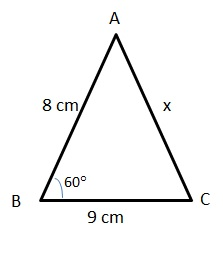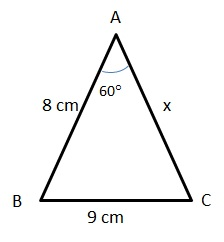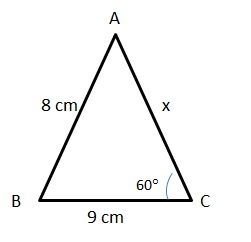# CAT Quantitative Aptitude Questions | Geometry Questions for CAT - Triangles

###### CAT Questions | CAT Geometry Questions | Triangles

The question is from the topic triangles. Two sides and one angle of a triangle are given we have to find out the possible values of the third side. CAT Geometry questions are heavily tested in CAT exam. Make sure you master Geometry problems. Apply the cosine rule here!

Question 33: The two sides of a triangle are 8 cm and 9 cm and one angle is 60. Which of the following can be the length of its third side?
I. √23 cm
II. √73 cm
III.(4.5 - √3.25) cm
IV. (4 + √33) cm
V. (9 + √13) cm

1. Only II and IV
2. Only I and III
3. Only I, II and V
4. Only II, III and IV

## Best CAT Coaching in Chennai

#### CAT Coaching in Chennai - CAT 2022Limited Seats Available - Register Now!

##### Method of solving this CAT Question from Triangles: There are three cases.

Case I:
Let x be the side opposite the angleBy cosine rule, x2 = 82 + 92 – 2*8*9*cos 60
x2 = 145 – 72 = 73
or x = √73
Case II:
Let 9 cm be the side opposite the angleBy cosine rule, 92 = 82 + x2 – 2*8*x*cos 60
17 = x2 - 8x
x2 - 8x - 17 = 0
or x = (8 +- √(64+68)) / 2
= 4 +- √33
Only 4 + √33
Case III:
Let 8 cm be the side opposite the angleBy cosine rule, 82 = 92 + x2 – 2*9*x*cos 60
-17 = x2 - 9x
x2 - 9x + 17 = 0
or x = (9 +- √(81−68)) / 2
= 4.5 +- (√13)/2
=4.5 +- √3.25

The question is "Choose the correct answer"

##### Hence, the answer is Only II, III and IV.

Choice D is the correct answer.

###### CAT Coaching in ChennaiCAT 2023

Classroom Batches Starting Now! @Gopalapuram

###### Best CAT Coaching in Chennai Introductory offer of 5000/-

Attend a Demo Class

## CAT Preparation Online | CAT Geometry questions Videos On YouTube

#### Other useful sources for Geometry Question | Geometry Triangles Circles Quadrilaterals Sample Questions

##### Where is 2IIM located?

2IIM Online CAT Coaching
A Fermat Education Initiative,
58/16, Indira Gandhi Street,
Kaveri Rangan Nagar, Saligramam, Chennai 600 093

##### How to reach 2IIM?

Phone: (91) 44 4505 8484
Mobile: (91) 99626 48484
WhatsApp: WhatsApp Now
Email: prep@2iim.com